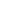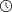Back to articles list- 8 minutes read

# What Does Cardinality Mean in a Database?

Cardinality in an SQL database isn’t just a number representing rows in a table. It also has an impact on query performance. Learn how database cardinality works in this article.

Cardinality is a term that originates from mathematics – more specifically, from relational algebra. But the term isn’t limited to the mathematical field; it also has implications in the world of databases. In this article, we will do a dive deep into this topic and explain cardinality in SQL databases and how it impacts your queries.

## Cardinality in Mathematics

First, let’s have some background information to better understand what cardinality means. Cardinality is a term originating from relational algebra, a subfield of mathematics. Cardinality is used as a measure for the number of elements in a set.

### What Is a Set?

A set is any collection of elements. This can be shapes, symbols, variables, or even lines. In mathematics (and relational algebra specifically), a set is usually a collection of numbers.

In the following image, we have two sets. Both of them have a cardinality of 7.As mentioned before, the set doesn’t have to contain necessarily only numbers in order for its cardinality to be calculated. Cardinality counts the number of elements contained in a set.

## Cardinality in Databases

Since the way relational databases work is based on relational algebra, many concepts of relational algebra are implemented in the internals of a working database engine.

For relational databases, the equivalent of a set is a simple table. As the set is a container for elements, a table is a container of rows, which can be viewed as “complex elements” (i.e. a row can have information stored in multiple columns).

However, in the domain of databases, the term cardinality has a slightly different definition. As opposed to counting the number of elements in a set, which would be equivalent to counting the number of rows in a table, cardinality in SQL represents the number of unique elements in that set.

But cardinality in databases isn’t limited to the entire table. Cardinality is determined at the column level. This is because, in databases, cardinality represents the number of unique elements. Because of this, each column can have a different cardinality, because it could have a different number of unique elements.

For example, a unique ID column will have distinct values for each row and its cardinality will be equal to the number of rows. However, a FirstName column can have a lower cardinality, because it is very likely that it will contain duplicate names.

Why is there a difference between the definition of cardinality in mathematics and cardinality in databases? Because in databases, cardinality is used in performance tuning; it aids the database engine in running queries as quickly as possible.

For example, let’s look in the `Person.Address` table from the AdventureWorks2019 database. Its schema is shown in the image below.If we count the number of unique values in `AddressLine1`, we can see that there are fewer unique values than rows in the table.We can compare it further with the `City` column and see how the cardinalities compare.Although the number of values for both `AddressLine1` and `City` is the same, the cardinality (number of unique values) is different for these two columns. We have 575 unique city names and 13,567 unique addresses in our table.

This scenario is one where we make the distinction between high cardinality and low cardinality columns. For example, in the columns where the number of unique values is the same or close to the number of total values in that column, we can say that the column has high cardinality. For columns where the number of unique values is much lower than the number of total values in the column, we can say that the column has low cardinality.

In our case, the high cardinality column is `AddressLine1`. This is because we have a lot of unique addresses, which is quite normal since almost everyone lives at a different address. However, because the `City` column isn’t so unique – thousands of people can live in the same city – we consider it a low cardinality column.

### Cardinality Impacts Query Performance

We mentioned that the cardinality information for a column is used by the database engine in the process of optimizing the SQL query. Let’s go into a bit more detail as to why that is the case.

We know that SQL is a declarative language and that the way you write the query isn’t necessarily the way it is executed behind the scenes by the database engine. The database engine syntactically analyzes your query and determines the tables and columns it needs to access, how to filter data, and how to group it if necessary. The order in which it does these operations is determined by the SQL Execution Plan.

In short, the Execution Plan tries to find the optimal path to return the data while using the least amount of server resources. To do this, it uses different data access operators. For example, look at SQL Server access operators; since relational databases are similar, the same data access operators exist in other database engines like MySQL or PostgreSQL.

Each of the data access operators is optimized to access, process, and return a different amount of data and rows. This is where the importance of cardinality comes in. Here’s a query that will be executed in SQL Server:The execution plan for this query looks like this:The execution plan shows that we access two indexes, one for each table containing the data we asked the query to return. If we hover the mouse cursor over the top Index Scan operator, we will have a pop-up showing us the number of estimated rows that will be read (based on cardinality) and the actual number of rows read at the end of the execution:An up-to-date cardinality in your columns, via statistics that are attached to each column, will give the database engine the best information so that it can generate the optimum path to return the data. Usually, the database engine updates the cardinality statistics automatically after a number of inserts and deletes, but you can also do this manually.

In this case, we can see that the statistics are up to date because the rows that the database engine estimated it will return are the same as the actual rows returned. If we add another city to filter by and be returned, like in the image below, this will also have a small impact on our query:If we look at the same data access operator, we will see that there is now a difference between the estimated and actual rows returned. That is because the cardinality estimation for the value of “Ottawa” was not accurate; it provided a higher estimate of actual rows than there actually existed in the table.In this case, the difference is only 6 rows. When the difference between estimated and actual rows is very large, the database engine might accidentally use a less optimal operator to return the data. In that case, we might have to wait longer for our query to execute or our server might consume more resources than optimal.

## Cardinality’s Impact During Data Modeling

One of the first places we should be thinking about cardinality is during data modeling. I will just briefly mention why cardinality is important during data modeling. If you want to go in-depth, see this article explaining cardinality in data modeling.

Whenever we start designing our database and we pass through the phases of data modeling, we eventually end up in the logical modeling phase. Here we split the logical entities into different entities, based on the principles of normalization.

By applying normalization, we try to reduce the redundancy of data by storing in different tables information that relates to the same logical entity. For example, a student can be enrolled in multiple courses, but his personal information never changes. We can represent this through a logical data model diagram like this one:The diagram represents that each student is enrolled in multiple courses and each course is presented to multiple students. There are several ways to represent this relationship, and there are different ERD notation standards you can use to model the data. One of the most popular – and the one we used above – is Crow’s Foot Notation.

In the context of data modeling, cardinality doesn’t refer to the number of rows in a column. Cardinality in data modeling isn’t a number, but a representation of a relationship. In this example, the cardinality of the `Student` table shows that a single row in this table is connected to many rows in the Student_Enrollments table; we call this a one-to-many relationship. A single row in the `Student` table has multiple correspondents in the `Student_Enrollments` table, which are identifiable by the `StudentID` column.

Similarly, the `Courses` table is linked with `Student_Enrollments` by the same one-to-many cardinality through the `CourseID` column. The one-to-many cardinality is one of the most common, but there are also one-to-one and many-to-many cardinalities.

All these cardinality definitions will go downstream to the next phase of the data modeling process, where we build out the physical data model. Our cardinality relationships will turn into foreign keys and constraints that will implement our data model. We won’t go into more detail about data modeling here,  since our goal was only to explain how cardinality is involved and represented in the modeling phase.

## Benefits of Cardinality in a Relational Database

At first, it might seem that cardinality in a database is a simple concept that represents the number of rows in a table. However, we can now see that it actually has a much larger impact.

It’s very important to determine the right cardinality when designing your database; this will impact the performance of your queries. If you determine the right cardinality and create the right data model, queries will automagically give you better performance with fewer resources used. You will not only have a more efficient database, but most likely a happier business team as well.

So, never forget about cardinality whenever you build, query, or performance tune a database.